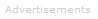# Definition of Charge

What is Electric Charge?
Q

Electric charge is a fundamental property of matter. Its symbol is Q or q.

Two Types of Charge

Charge comes in two varieties: positive and negative: positive charge (+q) is a property of protons; negative charge (-q) is a property of electrons. The charge on the proton is identical in size to that on the electron, but differs in sign.Electrically Neutral or Charged

If a body contains equal numbers of protons and electrons, then the positive charges are in balance with the negatives and the body is electrically neutral overall.

An excess of protons results in a net positive charge. An excess of electrons results in a net negative charge.

Charges in the Universe

It is believed the universe has equal numbers of protons and electrons and is therefore electrically neutral.

Units

The SI unit of charge is the coulomb, symbol C.

One coulomb is the amount of charge on 6.241509 x 1018 electrons (to seven significant figures).

Properties of Charge
Attraction/Repulsion

Alike charges repel. Therefore, positive repels positive, and negative repels negative.

Unalike charges attract: positive attracts negative, and vice versa.

Force of Attraction/Repulsion

The forces between two charges can be calculated using the inverse-square law known as Coulomb's law.

Consequences for Matter

Protons in the atomic nucleus repel one another. If the strong nuclear force is smaller than the force of electric repulsion, then the nucleus will be unstable: it will either not exist or it will be radioactive.

Electrons and protons attract one another electrically - these forces of attraction hold all atoms together and are responsible for all chemical bonds.

Charge Separation

In any locality, separation of electrical charges may exist, resulting in localized net positive or negative charge. Such a situation exists in atoms. Every individual atom is electrically neutral because it has the same number of protons and electrons. However, the positive charge is localized in the nucleus, while the negative charge occupies a much larger volume in the electron shells.

Electric Charge Separation by Ionization

Atoms can ionize to produce atom-sized particles that are electrically charged.

For example, Li atoms have a strong tendency to release an electron to become Li+ ions, while Cl2 molecules have a strong affinity for electrons:

Li   →   Li+   +  e-
Cl2  +  2e-  →   2Cl-

When brought together in a chemical reaction, these substances ionize, producing charged particles that attract one another:

2Li+   + Cl2  → 2Li+Cl-
Electric Potential

When you lift an object, you do work and the object gets gravitational potential energy. If you separate a positive and negative charge, you do work and the charges get electric potential energy: the amount of work you do depends on the amount of charge you separate and far you move the charges away from one another.

Electric potential, V, measures the amount of work done per unit of charge.

V = W/Q

The unit of electric potential is the volt (V). One volt is identical to one joule per coulomb.

Movement of Charge

A net flow of charge in a particular direction is called an electric current. Charges move in an electric potential field. In everyday terms, this means a voltage can produce a current.

Conductors are materials that charge can flow through. Materials that charge cannot flow through, or the flow is negligible, are termed insulators.

Current, symbol I, measures the rate that charge moves past any chosen point; it has units of coulombs per second, C s-1. The coulomb per second is usually called the amp or ampere, symbol A.

The movement of charge can result in energy losses - see electrical resistance and impedance - or no losses - see superconductivity.Search the Dictionary for More Terms